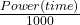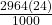## An oven at a 24 hr diner uses 24.7 A of electricity when it operates on a 120 V circuit. If electricity costs $0.080 per kWh, what would it Question An oven at a 24 hr diner uses 24.7 A of electricity when it operates on a 120 V circuit. If electricity costs$0.080 per kWh, what would it cost to run the stove while cooking food for one day? Assume the oven is on for 24 hrs at the same temperature.

in progress 0
6 months 2021-07-30T12:22:00+00:00 1 Answers 5 views 0

## Total cost to run the stove while cooking food for one day = $5.69 Explanation: An oven at a 24 hr diner uses 24.7 A of electricity when it operates on a 120 V circuit. Power consumed (P) = 24.7 (120) = 2964 W Hours of use per day = 24 Energy consumed per day === 71.136 kWh/day Electricity costs per kWh =$0.080
Total energy costs = 0.08 (71.136) = $5.69 Total cost to run the stove while cooking food for one day =$5.69